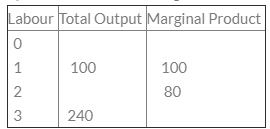# Test: Theory Of Production- 2

## 30 Questions MCQ Test Crash Course of Micro Economics -Class 12 | Test: Theory Of Production- 2

Description
Attempt Test: Theory Of Production- 2 | 30 questions in 30 minutes | Mock test for CA Foundation preparation | Free important questions MCQ to study Crash Course of Micro Economics -Class 12 for CA Foundation Exam | Download free PDF with solutions
QUESTION: 1

Solution:
QUESTION: 2

Solution:
QUESTION: 3

### A production function is defined as the relationship between ________.

Solution:
QUESTION: 4

Average Fixed Cost = Rs. 20 Quantity Produces = 10 units What will be the Average Fixed Cost of 20th unit?

Solution:
QUESTION: 5

Increasing returns to scale occurs due to:

Solution:

An increasing returns to scale occurs when the output increases by a larger proportion than the increase in inputs during the production process. For example, if input is increased by 3 times, but output increases by 3.75 times, then the firm or economy has experienced an increasing returns to scale.

QUESTION: 6

Functions of the entrepreneur are:

Solution:
QUESTION: 7

External Economies of scale are obtained by

Solution:
QUESTION: 8

In the first stage of law of variable proportions, total product increases at the ______

Solution:
QUESTION: 9

Which of the following is the reason of the working of law of increasing returns?

Solution:
QUESTION: 10

During IInd stage of law of Diminishing returns:

Solution:
QUESTION: 11

Labour force wants more_______.

Solution:
QUESTION: 12

Increase in all input leading to less than proportional increase in output is called ______:

Solution:
QUESTION: 13

Who has given the concept of Innovative Entrepreneurship?

Solution:
QUESTION: 14

Which function shows relationship between input and output?

Solution:
QUESTION: 15

The Law of Diminishing Returns is applicable in _________.

Solution:
QUESTION: 16

In the first stage of law of variable proportions, total product increases at the ______

Solution:
QUESTION: 17

An Entrepreneur undertakes which one of the following functions?

Solution:
QUESTION: 18

In Cobb-Douglas production function, two inputs are

Solution:
QUESTION: 19

Consider the following table:What is the total output, when 2 labour are employed?

Solution:
QUESTION: 20

Production function is

Solution:
QUESTION: 21

At the point of inflexion, the marginal product is:

Solution:
QUESTION: 22

Production activity in the short period is analysed with the help of

Solution:
QUESTION: 23

Increase in all input leading to less than proportional increase in output is called ______:

Solution:
QUESTION: 24

The three broad types of productive resources are:

Solution:
QUESTION: 25

External economics are enjoyed:

Solution:

The correct option is Option B.

Industry refers to a number of markets grouped together. So when an industry expands, there are a lot of sectors that are benefitted. All the firms in the economy get external economies i.e., they get the benefit of large scale business and they tend to grow further.

QUESTION: 26

Labour force wants more_______.

Solution:
QUESTION: 27

The function of an entrepreneur is:

Solution:
QUESTION: 28

Law of diminishing returns is applicable in _________

Solution:
QUESTION: 29

If a firm’s output is zero, then

Solution:
QUESTION: 30

Average Fixed Cost = Rs. 20
Quantity Produced = 10 units
What will be the Average Fixed Cost of 20th unit?

Solution:

AFC =TFc/tu
where, Afc = RS 20 TU=10 Units
TfC=20*10= 200 (cross multi fication)
afc = tfc /tu
200/20= 10 ansUse Code STAYHOME200 and get INR 200 additional OFF Use Coupon Code